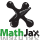JavaScript calculusCalculus, the old-fashioned way

Zeno and Archimedes are having a drink when they see an attractive lady across the bar. Archimedes gets up to go talk to her. Zeno says, “Why bother? You will first need to cover half the distance, and then half the remaining distance, and so on, so you will never get there.” Archimedes says, “Maybe not, but I’ll get close enough.”

As a student, it is easy to get the impression that calculus is a magnificent system of integrals and derivatives that someone somewhere has validated with rigorous limit proofs. In the real world, not all datasets are able to be represented by elementary functions, and some seemingly-straightforward problems (such as the circumference of an ellipse !) have no elementary solution. So, mathematical purists notwithstanding, calculus must sometimes be performed with infinitesimals and brute-force numeric methods. (Plus, infinitesimals are a lot easier to understand! Plus, rigorous or not, they work! Must side with Leibniz here.)

To approximate the tangent to a curve at a point (the derivative), find the slope of the line that connects closely-spaced points on either side (the symmetric difference quotient):$$% MathJax is loading . . . . \quad m = (y_1 - y_{–1}) / (x_1 - x_{–1})$$

The tangent line:

$$\quad y = m\,(x - x_0) + y_0$$

To approximate the area under a curve (the integral), partition it into narrow trapezoids and find the sum of the areas (the method of exhaustion).

The area of each trapezoid is the area of the rectangle plus the area of the triangle on top.
It is equivalent to the width × the average of the heights:

$$\quad A \approx (x_1 - x_0) \, ((y_1 + y_0) / 2)$$

The arc length is approximated by the diagonal:

$$\quad s \approx \sqrt { (x_1 - x_0)^2 + (y_1 - y_0)^2 }$$

The narrower the trapezoids, the closer the approximation.

To approximate area using polar coordinates, partition the figure into narrow triangles.

If the incremental angle $$\theta_1 - \theta_0 = \alpha$$ is very small, the triangles may be treated as if they were right triangles with both hypotenuse and long side of length $$r$$, and short side of length $$r \, \textrm{sin} \, \alpha$$.

The area of each right triangle would be approximately $$( r \cdot r \, \textrm{sin} \, \alpha ) / 2$$.

The area of the segment of the figure may be more closely approximated by averaging the areas of the $$r_0$$ and $$r_1$$ right triangles, as with the trapezoids above; for very small α,

$$\quad A \approx ( r_0 \, r_1 \, \textrm{sin} \, \alpha ) / 2$$

The arc length is approximated by the $$r_0 \, – \, r_1$$ diagonal:

$$\quad s \approx \sqrt { (r_1 - r_0)^2 + r_0 \, r_1 \, (\textrm{sin} \, \alpha) ^2 }$$

or, in Cartesian form:

$$\quad s \approx \sqrt { (r_1 \, \textrm{cos} \, \theta_1 - r_0 \, \textrm{cos} \, \theta_0)^2 + (r_1 \, \textrm{sin} \, \theta_1 - r_0 \, \textrm{sin} \, \theta_0)^2 }$$

The narrower the angle, the closer the approximation.

The above may be applied to three-dimensional figures as well, by summing the areas and volumes of infinitesimal strips and slices.

To test the accuracy of the above, we can apply it to figures whose math is well-known.

Begin with a parabola, $$y = x^2$$.

Let $$x_0 =$$ .  The slope of the tangent at $$x_0$$ is the derivative

$$\quad \displaystyle \frac{d}{dx} x^2 = 2x_0 =$$

Using an x increment of , the slope of the line connecting points on either side is .

Let $$x_1 =$$ .  The area under the curve from 0 to $$x_1$$ is the integral

$$\quad \displaystyle \int_{0}^{x_1} x^2 \,dx= \frac{{x_1}^3}{3} =$$

The calculated area by trapezoids is .

Now for an ellipse, where $$a = 16, ~ b = 5, ~ c = \sqrt { a^2 - b^2 },$$ and $$\displaystyle e = \frac {c}{a}.$$
When the origin is at one focus, $$~ \displaystyle r = \frac {a\,(1 - e^2)}{1 - e\,\textrm{cos}\theta}$$

Let θ0 = °. The slope of the tangent at θ0 is

$$\displaystyle \quad \frac {– b^2\,(x-c)}{a^2\,y} =$$

Using an angle increment of radians, the slope of the line connecting points on either side is .

The area of an ellipse is $$\pi a b =$$
The calculated area by triangles is .

The circumference of an ellipse has no elementary solution, but may be calculated to any degree of precision by the following formula:

$$\quad \displaystyle C = \pi \, (a+b) \left[ 1+\sum _{n=1}^{\infty } \left( {\frac {(2n-1)!!}{2^{n}n!}} \right) ^{2}{\frac {h^{n}}{(2n-1)^{2}}} \right],$$ where $$\displaystyle h=(a-b)^{2}/(a+b)^{2}$$

Taken to greater than 15 significant figures, the circumference of the ellipse is .
The calculated circumference by $$r_0 \, – \, r_1$$ segments is .

Note that infinitesimal methods may be used to find the tangent to any ellipse, such as one rotated at an arbitrary angle; and they may be used to find any segment of area or circumference.

Now for something a lot more practical. What is the area of the shaded slice of pizza?

The pizza radius is 1 and the slice dimensions are 13 × 13.

The equation for the top half of the pizza is $$y = \sqrt{1 - x^2} .$$

Using an x increment of , the calculated area by trapezoids is , or  % of a full slice.

The length of the crust by $$r_0 \, – \, r_1$$ segments is .

Starting the year in which the owner turns 72, retirement accounts must make an annual required minimum distribution based on life expectancy tables published by the Internal Revenue Service. The graph at right shows what happens to a $100,000 account with the specified growth rate as RMD’s are withdrawn in monthly installments. growth rate: 4.0 % age: 84 | month: February The rate of change in the year the owner turns is$  per year.
The area under the curve (total value × time) is \$ .